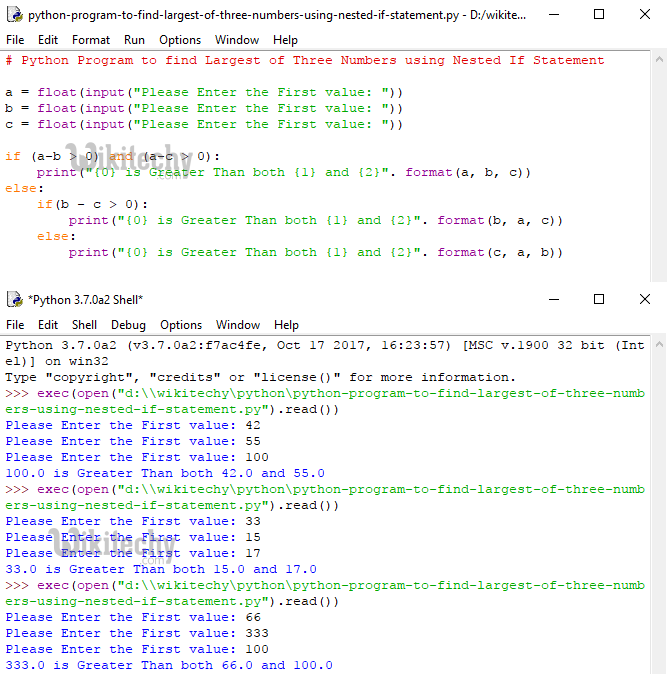# python tutorial - Python Program to Find Largest of Three numbers - learn python - python programming

• Although We have many approaches to find largest number among three numbers.
• How to write a Python program to find largest of three numbers using Elif Statement and Python Nested If.Learn Python - Python tutorial - Python Program to Find Largest of Three numbers - Python examples - Python programs

## Python Program to find Largest of Three numbers using Elif Statement

• This program helps the user to enter three different values and then it will find the largest number among that three numbers using Python Elif Statement

## Analysis

• Following statements ask the user to enter three numbers and stores the user entered values in variables a, b and c
• First if condition check whether a is greater than b and a is greater than c.
• If both of these are True then following print statement will be displayed (a is greater than both b, c).
• First Elif statement check whether b is greater than a and b is greater than c.
• If both of these are True then following print statement will be displayed ( b is greater than both a, c).
• Second Elif statement check whether c is greater than a and c is greater than b.
• If both of these are True then following print statement will be displayed ( c is greater than both a, b).
• If all the above conditions fail, it means they are equal.

## Output:

• First, we entered the values a = 13, b = 5, c= 7 and Next, we entered the values a = 25, b = 30, c= 15 and Next, we entered the values a = 60, b = 50, c= 90 and Finally, we entered the values a = 7, b = 7, c= 7Learn Python - Python tutorial - Python Program to Find Largest of Three numbers - Python examples - Python programs

## Python Program to find Largest of Three numbers using Nested If Statement

• This program helps the user to enter three different values and then it will find the largest number among that three numbers using Python Nested If.

## Analysis

• Following statements ask the user to enter three numbers and stores the user entered values in variables a, b and c
• First if condition check whether a-b is greater than 0 and a-c is greater than 0.
• If we subtract small number from big number then this condition fail, otherwise it will be True.
• If this condition is True then a is greater than both b, c.
• Else statement will execute when the first If condition is False so, there is no need to check for a value.
• In the Else statement we are inserting one more if condition (Nested IF) to check whether b-c is greater than 0.
• If this condition is True then b is greater than both a, c.
• Else c is greater than both a, b.

## OutputLearn Python - Python tutorial - Python Program to find Largest of Three numbers using Elif Statement - Python examples - Python programs